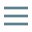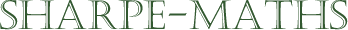# Glossary of terms

## N

•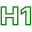### Natural numbers ($\Bbb{N}$)

•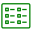The Natural numbers are the counting numbers. The positive whole numbers. The first Natural number is 1 and there are an infinite number of them.

•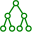$3 \in\Bbb{N}$

3 is a member of the set of Natural numbers

•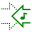•### Negative numbers

•Negative numbers are any numbers which exist on the number line, and which are to the left of zero.They are extremely useful when considering concepts like debt in Economics, height below sea level in Geography, and similar ideas.

•••### Number line

•The Number line is an imaginary line which stretches left to right, from $- \infty$  to  $+\infty$.

It can be a very useful visual aid for any numerical work in mathematics.•••### Numbers

•• Natural numbers ($\Bbb{N}$): Positive whole numbers
• Integers ($\Bbb{Z}$): Positive & Negative whole numbers & Zero
• Rational numbers ($\Bbb{Q}$): All numbers which can be written in fractional form. (i.e. An integer divided by another integer.
• Real numbers($\Bbb{R}$): All numbers on the number line. Real numbers include the irrational numbers.
• Complex numbers ($\Bbb{C}$): All numbers including those with imaginary parts.

The different types of numbers and their relationship to one another is shown in the Venn Diagram below.For those students studying at pre-16 level, only need to concern yourselves about the inner 4 ellipses, out to the Real numbers.

Post-16 students may require Complex numbers as well, though, in the UK, only when studying Further Mathematics.

••